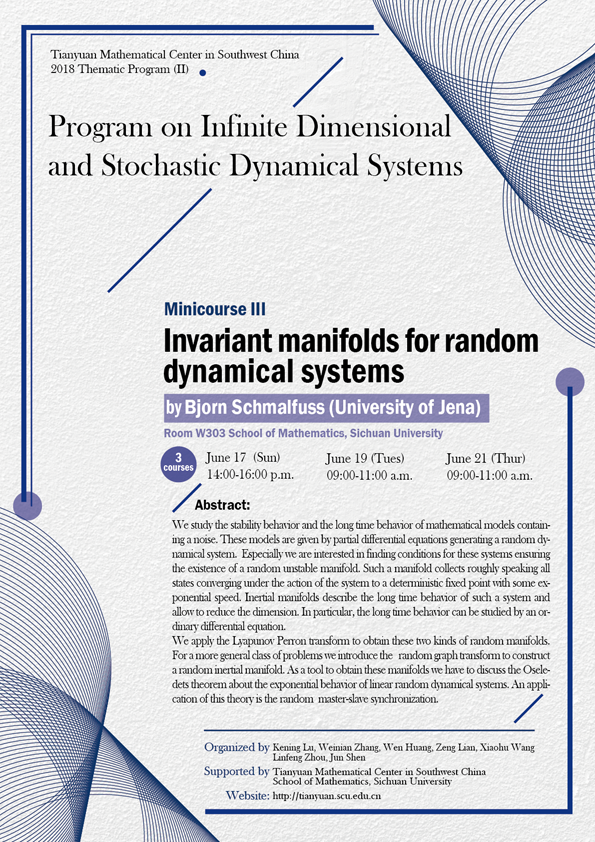2018 Thematic Program (II)

### Invariant manifolds for random dynamical systems

#### W303  School of Mathematics, Sichuan University#### SPEAKER

Bjorn Schmalfuss (University of Jena)

#### ABSTRCT

We study the stability behavior and the long time behavior of mathematical models containing a noise. These models are given by partial differential equations generating a random dynamical system. Especially we are interested in finding conditions for these systems ensuring the existence of a random unstable manifold. Such a manifold collects roughly speaking all states converging under the action of the system to a deterministic fixed point with some exponential speed. Inertial manifolds describe the long time behavior of such a system and allow to reduce the dimension. In particular, the long time behavior can be studied by an ordinary differential equation.

We apply the Lyapunov Perron transform to obtain these two kinds of random manifolds. For a more general class of problems we introduce the random graph transform to construct a random inertial manifold. As a tool to obtain these manifolds we have to discuss the Oseledets theorem about the exponential behavior of linear random dynamical systems. An application of this theory is the random master-slave synchronization.

#### ORGANIZERS

Kening Lu (Sichuan University)

Weinian Zhang (Sichuan University)

Wen Huang (Sichuan University)

Zeng Lian (Sichuan University)

Xiaohu Wang (Sichuan University)

Linfeng Zhou (Sichuan University)

Jun Shen (Sichuan University)

#### SUPPORTED BY

Tianyuan Mathematical Center in Southwest China

School of Mathematics, Sichuan University